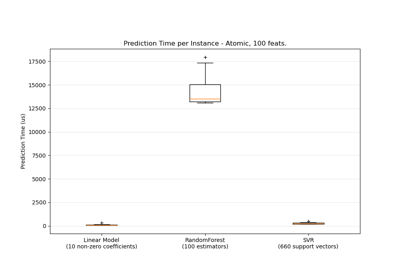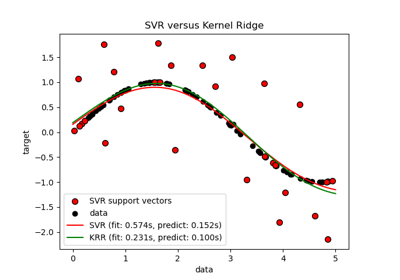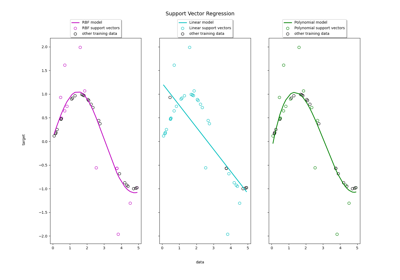# sklearn.svm.SVR¶

class sklearn.svm.SVR(*, kernel='rbf', degree=3, gamma='scale', coef0=0.0, tol=0.001, C=1.0, epsilon=0.1, shrinking=True, cache_size=200, verbose=False, max_iter=-1)[source]

Epsilon-Support Vector Regression.

The free parameters in the model are C and epsilon.

The implementation is based on libsvm. The fit time complexity is more than quadratic with the number of samples which makes it hard to scale to datasets with more than a couple of 10000 samples. For large datasets consider using LinearSVR or SGDRegressor instead, possibly after a Nystroem transformer or other Kernel Approximation.

Read more in the User Guide.

Parameters:
kernel{‘linear’, ‘poly’, ‘rbf’, ‘sigmoid’, ‘precomputed’} or callable, default=’rbf’

Specifies the kernel type to be used in the algorithm. If none is given, ‘rbf’ will be used. If a callable is given it is used to precompute the kernel matrix.

degreeint, default=3

Degree of the polynomial kernel function (‘poly’). Must be non-negative. Ignored by all other kernels.

gamma{‘scale’, ‘auto’} or float, default=’scale’

Kernel coefficient for ‘rbf’, ‘poly’ and ‘sigmoid’.

• if gamma='scale' (default) is passed then it uses 1 / (n_features * X.var()) as value of gamma,

• if ‘auto’, uses 1 / n_features

• if float, must be non-negative.

Changed in version 0.22: The default value of gamma changed from ‘auto’ to ‘scale’.

coef0float, default=0.0

Independent term in kernel function. It is only significant in ‘poly’ and ‘sigmoid’.

tolfloat, default=1e-3

Tolerance for stopping criterion.

Cfloat, default=1.0

Regularization parameter. The strength of the regularization is inversely proportional to C. Must be strictly positive. The penalty is a squared l2 penalty.

epsilonfloat, default=0.1

Epsilon in the epsilon-SVR model. It specifies the epsilon-tube within which no penalty is associated in the training loss function with points predicted within a distance epsilon from the actual value. Must be non-negative.

shrinkingbool, default=True

Whether to use the shrinking heuristic. See the User Guide.

cache_sizefloat, default=200

Specify the size of the kernel cache (in MB).

verbosebool, default=False

Enable verbose output. Note that this setting takes advantage of a per-process runtime setting in libsvm that, if enabled, may not work properly in a multithreaded context.

max_iterint, default=-1

Hard limit on iterations within solver, or -1 for no limit.

Attributes:
class_weight_ndarray of shape (n_classes,)

Multipliers of parameter C for each class. Computed based on the class_weight parameter.

Deprecated since version 1.2: class_weight_ was deprecated in version 1.2 and will be removed in 1.4.

coef_ndarray of shape (1, n_features)

Weights assigned to the features when kernel="linear".

dual_coef_ndarray of shape (1, n_SV)

Coefficients of the support vector in the decision function.

fit_status_int

0 if correctly fitted, 1 otherwise (will raise warning)

intercept_ndarray of shape (1,)

Constants in decision function.

n_features_in_int

Number of features seen during fit.

New in version 0.24.

feature_names_in_ndarray of shape (n_features_in_,)

Names of features seen during fit. Defined only when X has feature names that are all strings.

New in version 1.0.

n_iter_int

Number of iterations run by the optimization routine to fit the model.

New in version 1.1.

n_support_ndarray of shape (1,), dtype=int32

Number of support vectors for each class.

shape_fit_tuple of int of shape (n_dimensions_of_X,)

Array dimensions of training vector X.

support_ndarray of shape (n_SV,)

Indices of support vectors.

support_vectors_ndarray of shape (n_SV, n_features)

Support vectors.

NuSVR

Support Vector Machine for regression implemented using libsvm using a parameter to control the number of support vectors.

LinearSVR

Scalable Linear Support Vector Machine for regression implemented using liblinear.

References

Examples

>>> from sklearn.svm import SVR
>>> from sklearn.pipeline import make_pipeline
>>> from sklearn.preprocessing import StandardScaler
>>> import numpy as np
>>> n_samples, n_features = 10, 5
>>> rng = np.random.RandomState(0)
>>> y = rng.randn(n_samples)
>>> X = rng.randn(n_samples, n_features)
>>> regr = make_pipeline(StandardScaler(), SVR(C=1.0, epsilon=0.2))
>>> regr.fit(X, y)
Pipeline(steps=[('standardscaler', StandardScaler()),
('svr', SVR(epsilon=0.2))])


Methods

 fit(X, y[, sample_weight]) Fit the SVM model according to the given training data. Get metadata routing of this object. get_params([deep]) Get parameters for this estimator. Perform regression on samples in X. score(X, y[, sample_weight]) Return the coefficient of determination of the prediction. set_fit_request(*[, sample_weight]) Request metadata passed to the fit method. set_params(**params) Set the parameters of this estimator. set_score_request(*[, sample_weight]) Request metadata passed to the score method.
property coef_

Weights assigned to the features when kernel="linear".

Returns:
ndarray of shape (n_features, n_classes)
fit(X, y, sample_weight=None)[source]

Fit the SVM model according to the given training data.

Parameters:
X{array-like, sparse matrix} of shape (n_samples, n_features) or (n_samples, n_samples)

Training vectors, where n_samples is the number of samples and n_features is the number of features. For kernel=”precomputed”, the expected shape of X is (n_samples, n_samples).

yarray-like of shape (n_samples,)

Target values (class labels in classification, real numbers in regression).

sample_weightarray-like of shape (n_samples,), default=None

Per-sample weights. Rescale C per sample. Higher weights force the classifier to put more emphasis on these points.

Returns:
selfobject

Fitted estimator.

Notes

If X and y are not C-ordered and contiguous arrays of np.float64 and X is not a scipy.sparse.csr_matrix, X and/or y may be copied.

If X is a dense array, then the other methods will not support sparse matrices as input.

Get metadata routing of this object.

Please check User Guide on how the routing mechanism works.

Returns:

A MetadataRequest encapsulating routing information.

get_params(deep=True)[source]

Get parameters for this estimator.

Parameters:
deepbool, default=True

If True, will return the parameters for this estimator and contained subobjects that are estimators.

Returns:
paramsdict

Parameter names mapped to their values.

property n_support_

Number of support vectors for each class.

predict(X)[source]

Perform regression on samples in X.

For an one-class model, +1 (inlier) or -1 (outlier) is returned.

Parameters:
X{array-like, sparse matrix} of shape (n_samples, n_features)

For kernel=”precomputed”, the expected shape of X is (n_samples_test, n_samples_train).

Returns:
y_predndarray of shape (n_samples,)

The predicted values.

score(X, y, sample_weight=None)[source]

Return the coefficient of determination of the prediction.

The coefficient of determination $$R^2$$ is defined as $$(1 - \frac{u}{v})$$, where $$u$$ is the residual sum of squares ((y_true - y_pred)** 2).sum() and $$v$$ is the total sum of squares ((y_true - y_true.mean()) ** 2).sum(). The best possible score is 1.0 and it can be negative (because the model can be arbitrarily worse). A constant model that always predicts the expected value of y, disregarding the input features, would get a $$R^2$$ score of 0.0.

Parameters:
Xarray-like of shape (n_samples, n_features)

Test samples. For some estimators this may be a precomputed kernel matrix or a list of generic objects instead with shape (n_samples, n_samples_fitted), where n_samples_fitted is the number of samples used in the fitting for the estimator.

yarray-like of shape (n_samples,) or (n_samples, n_outputs)

True values for X.

sample_weightarray-like of shape (n_samples,), default=None

Sample weights.

Returns:
scorefloat

$$R^2$$ of self.predict(X) w.r.t. y.

Notes

The $$R^2$$ score used when calling score on a regressor uses multioutput='uniform_average' from version 0.23 to keep consistent with default value of r2_score. This influences the score method of all the multioutput regressors (except for MultiOutputRegressor).

set_fit_request(*, sample_weight: Union[bool, None, str] = '$UNCHANGED$') SVR[source]

Request metadata passed to the fit method.

Note that this method is only relevant if enable_metadata_routing=True (see sklearn.set_config). Please see User Guide on how the routing mechanism works.

The options for each parameter are:

• True: metadata is requested, and passed to fit if provided. The request is ignored if metadata is not provided.

• False: metadata is not requested and the meta-estimator will not pass it to fit.

• None: metadata is not requested, and the meta-estimator will raise an error if the user provides it.

• str: metadata should be passed to the meta-estimator with this given alias instead of the original name.

The default (sklearn.utils.metadata_routing.UNCHANGED) retains the existing request. This allows you to change the request for some parameters and not others.

New in version 1.3.

Note

This method is only relevant if this estimator is used as a sub-estimator of a meta-estimator, e.g. used inside a Pipeline. Otherwise it has no effect.

Parameters:
sample_weightstr, True, False, or None, default=sklearn.utils.metadata_routing.UNCHANGED

Metadata routing for sample_weight parameter in fit.

Returns:
selfobject

The updated object.

set_params(**params)[source]

Set the parameters of this estimator.

The method works on simple estimators as well as on nested objects (such as Pipeline). The latter have parameters of the form <component>__<parameter> so that it’s possible to update each component of a nested object.

Parameters:
**paramsdict

Estimator parameters.

Returns:
selfestimator instance

Estimator instance.

set_score_request(*, sample_weight: Union[bool, None, str] = '$UNCHANGED$') SVR[source]

Request metadata passed to the score method.

Note that this method is only relevant if enable_metadata_routing=True (see sklearn.set_config). Please see User Guide on how the routing mechanism works.

The options for each parameter are:

• True: metadata is requested, and passed to score if provided. The request is ignored if metadata is not provided.

• False: metadata is not requested and the meta-estimator will not pass it to score.

• None: metadata is not requested, and the meta-estimator will raise an error if the user provides it.

• str: metadata should be passed to the meta-estimator with this given alias instead of the original name.

The default (sklearn.utils.metadata_routing.UNCHANGED) retains the existing request. This allows you to change the request for some parameters and not others.

New in version 1.3.

Note

This method is only relevant if this estimator is used as a sub-estimator of a meta-estimator, e.g. used inside a Pipeline. Otherwise it has no effect.

Parameters:
sample_weightstr, True, False, or None, default=sklearn.utils.metadata_routing.UNCHANGED

Metadata routing for sample_weight parameter in score.

Returns:
selfobject

The updated object.

## Examples using sklearn.svm.SVR¶Prediction Latency

Prediction LatencyComparison of kernel ridge regression and SVR

Comparison of kernel ridge regression and SVRSupport Vector Regression (SVR) using linear and non-linear kernels

Support Vector Regression (SVR) using linear and non-linear kernels